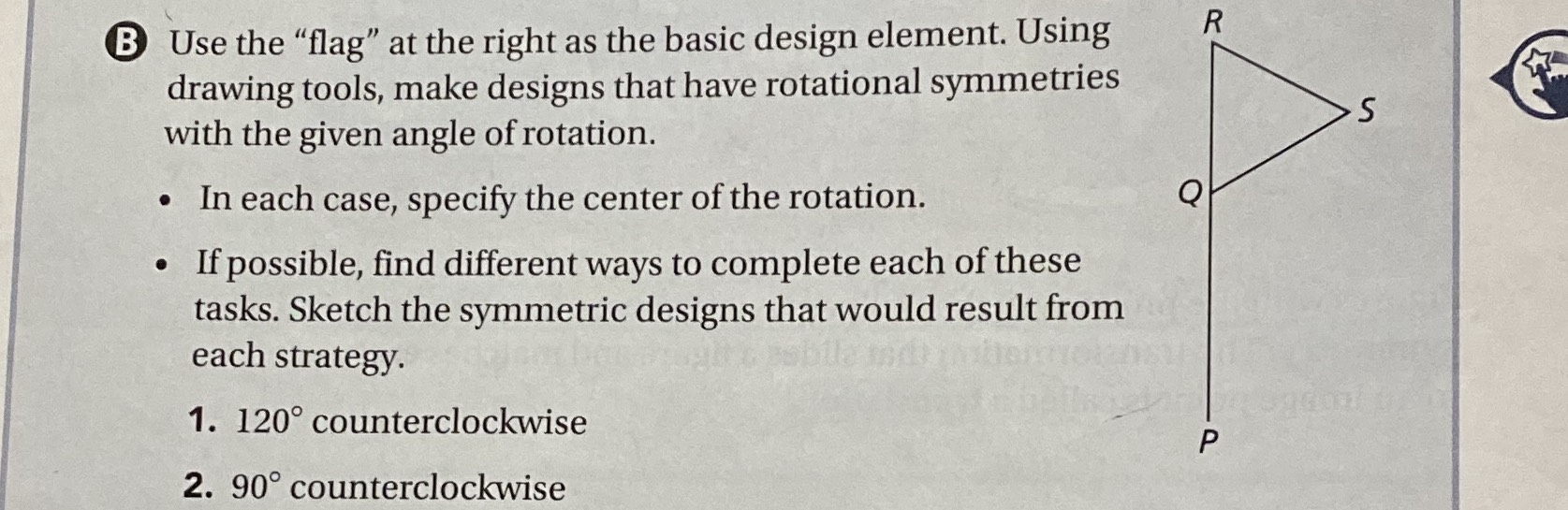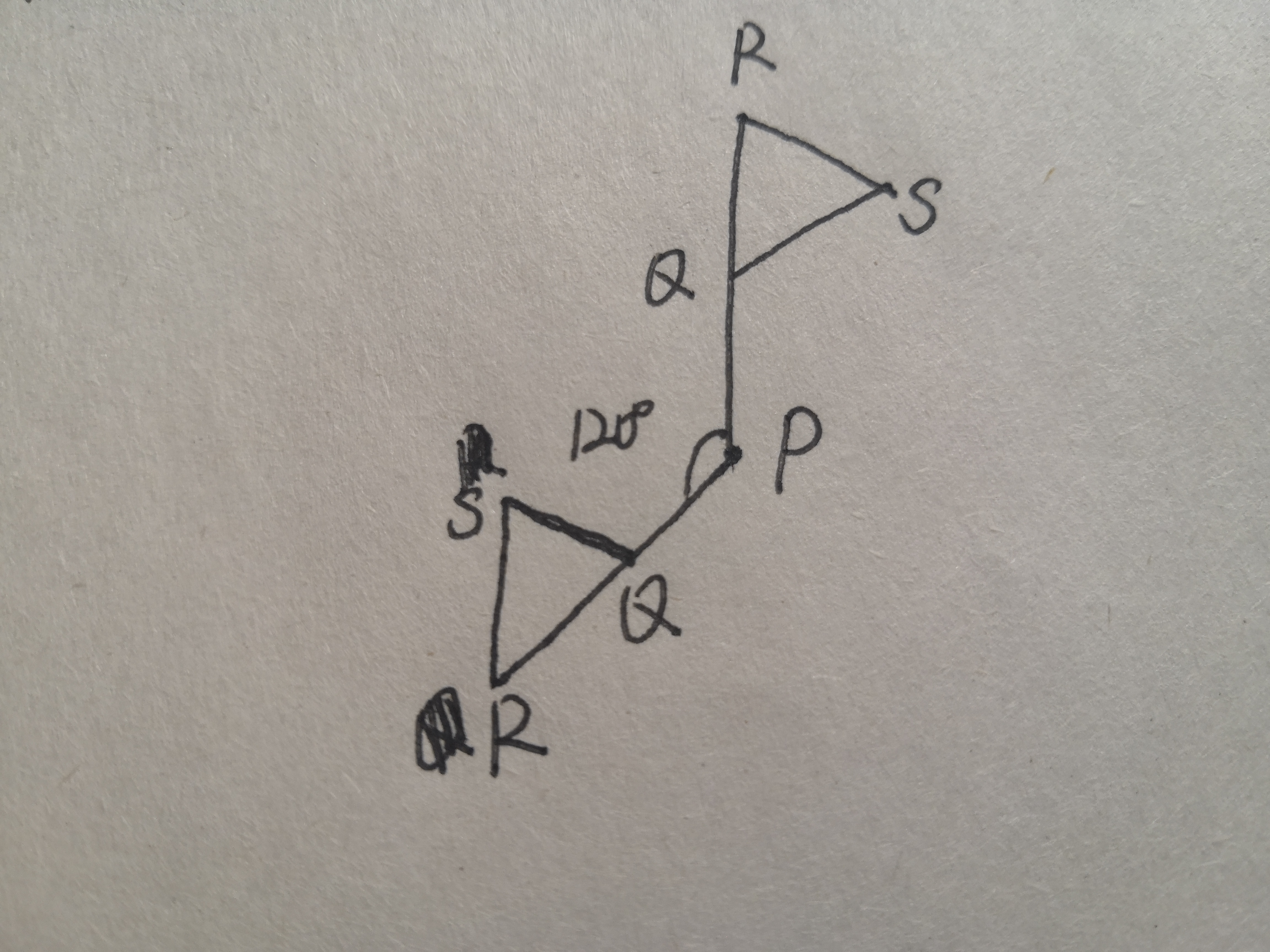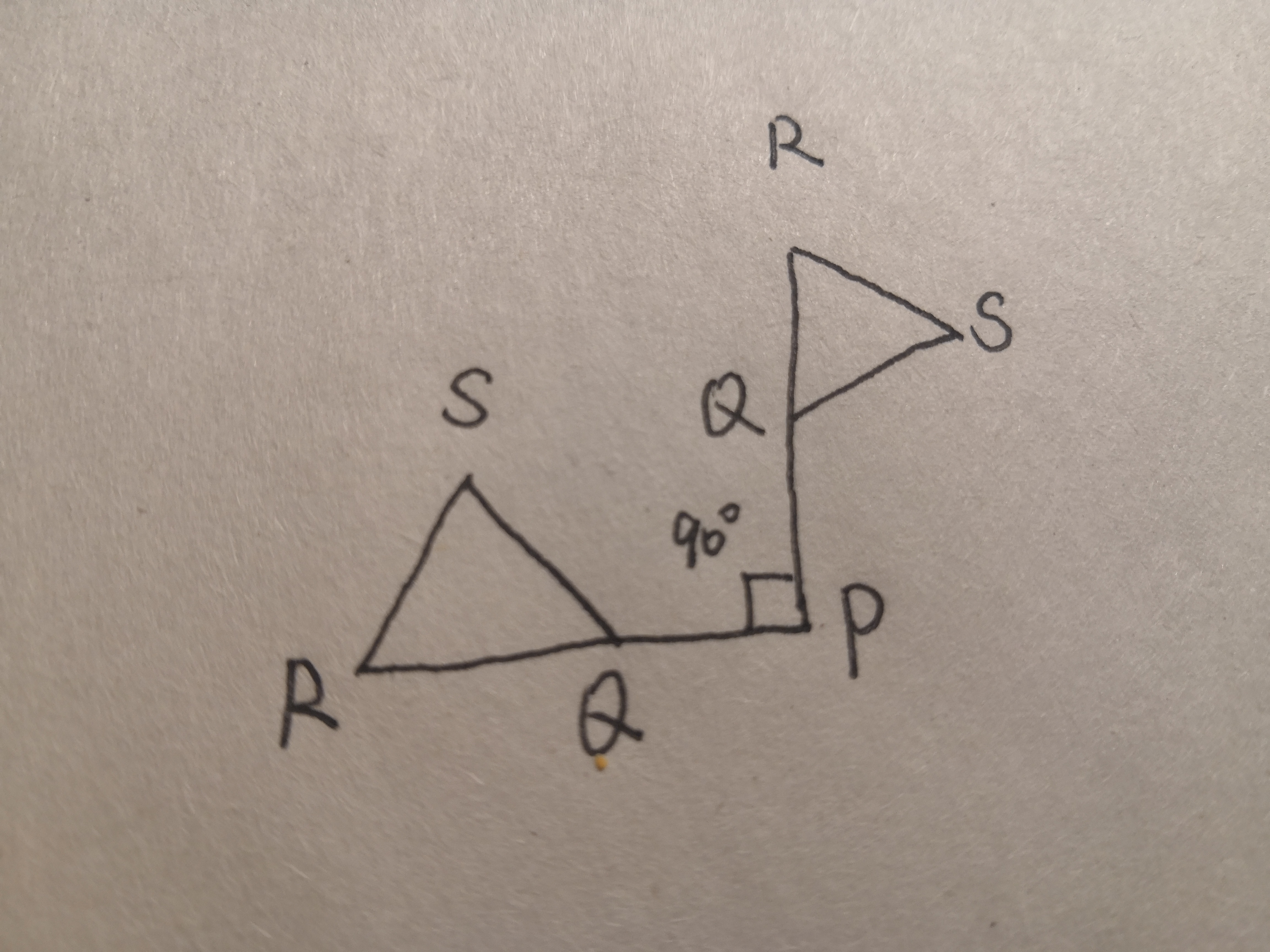### Still have math questions?

Trigonometry
QuestionUse the "flag" at the right as the basic design element. Using drawing tools, make designs that have rotational symmetries with the given angle of rotation.

1. In each case, specify the center of the rotation.

2. If possible, find different ways to complete each of these each strategy.

1). $$120 ^ { \circ }$$ counterclockwise

2). $$90 ^ { \circ }$$ counterclockwise

1,